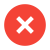﻿ Pressure Difference - Delta P - Performance Monitoring Water Treatment

# Pressure Difference – Delta P (ΔP)

Delta P (ΔP), pressure difference or differential pressure usually refers in the technical world to the drop of pressure in a piping system, a heat exchanger or another machine, where a liquid is passing through.
The delta symbol Δ, is the fourth letter in the Greek and the Coptic alphabet. Delta / Δ is used as a mathematical symbol, which represents the „difference“ between two values.

ΔP stands for the difference between two measured pressure values. This can be measured either at different times/dates or at different positions in a system. The measurement at different times, can be a continuous measurement from day to day to see a trend as the ΔP develops over a longer period of time. Measurement at different positions in a system, i.e. you compare the pressure at the input with the pressure at the output of a machine. And get the pressure drop - Delta P.
The meassurement of a pressure, either an analog pressure gauge is used or an electrical sensor, which delivers its value to a central data system.

In a piping system or a heat exchanger with a moving fluid, the pressure usually drops due to friction. The friction happens in between the water and the contacting surfaces, e.g. the pipe wall. As higher the differnce, as more likely there is fouling in the system.
This pressure drop will increase as more incrustations are in the way of flow. The deposits in a water system are caused by limescale, suspended solids, biological growth or other kinds of fouling. The deposits build up in the pipe and interfere with the water flow. Therefore ΔP can be used to measure the flow resistance. One can calculate the amount of deposits in the pipe or heat exchanger. The unit for the pressure is given in millipascal / mPa. Which indicates the force acting on a surface.

The ΔP in the heat exchanger to the left can be calculated very simple. Subtract the inlet pressure (P1) at the point B, from the outlet pressure (P2) at the exit A and you will get Delta P.

The equation for the pressure difference is: ΔP = P2 – P1

It is obvious that a high concentration of deposits, in pipe or exchanger, lead to a high pressure drop, ΔP. If there are deposits the water can't flow free. Hence the outlet pressure is much lower than the inlet pressure.

## Examples about delta P the differential pressure

If you are sitting in an airplane and drink from a plastic bottle, then the pressure in the bottle is the same as in the airplane. Once you have landed you will notice that the bottle is pushed inwards. That's because the pressure in the plane is much lower than the atmospheric pressure at the ground. This pressure difference can be seen on the bottle. The delta P would be the difference in between the lower pressure in the airplane and the normal pressure on the ground.

We at Merus use delta P for the performance monitoring. After the installation of Merus Rings the delta P decreases, if all works well to its design level.